Image Types & Data Classes

Image Types

There are five types of images in MATLAB.
Grayscale: A grayscale image M pixels tall and N pixels wide is represented as a matrix of double datatype of size [M X N]. Element values (e.g., MyImage(m,n)) denote the pixel grayscale intensities in [0,1] with 0=black and 1=white.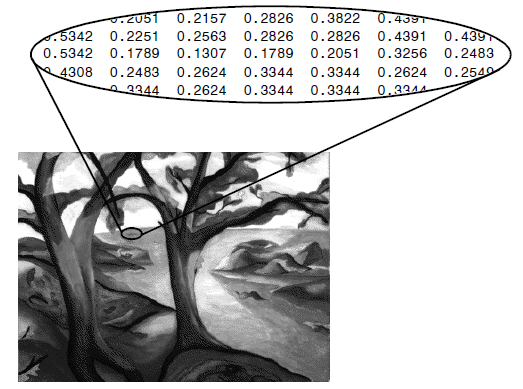Truecolor RGB: A truecolor red-green-blue (RGB) image is represented as a three-dimensional M×N×3 double matrix. Each pixel has red, green, blue components along the third dimension with values in [0,1], for example, the color components of pixel (m,n) are MyImage(m,n,1) = red, MyImage(m,n,2) = green, MyImage(m,n,3) = blue. If each of these components has a range 0–255, this gives a total of 256*3 different possible colors. Such an image is a “stack” of three matrices; representing the red, green and blue values for each pixel. This means that for every pixel there are 3 corresponding values. True color image may be of type Int or Double.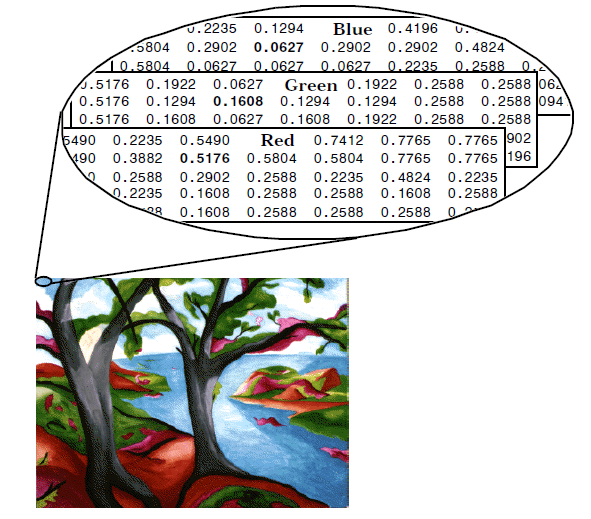Indexed: Indexed (paletted) images are represented with an index matrix of size M×N and a colormap matrix of size K×3. The image has in total  K different colors. The colormap holds all colors used in the image and the index matrix represents the pixels by referring to colors in the colormap. For example, if the 22nd color is magenta MyColormap(22,:) = [1,0,1], then MyImage(m,n) = 22 is a magenta-colored pixel.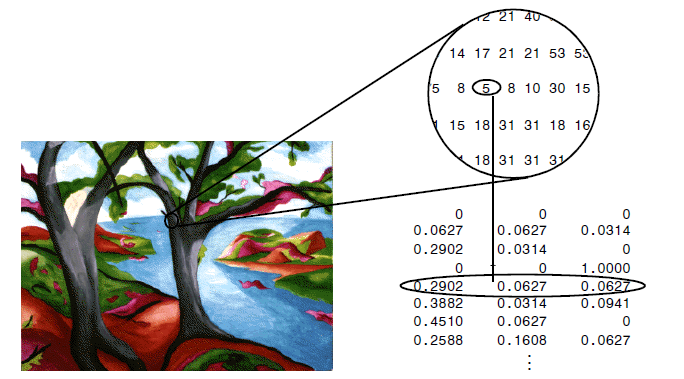Binary: A binary image is represented by an M×N logical matrix where pixel values are 1 (true) or 0 (false).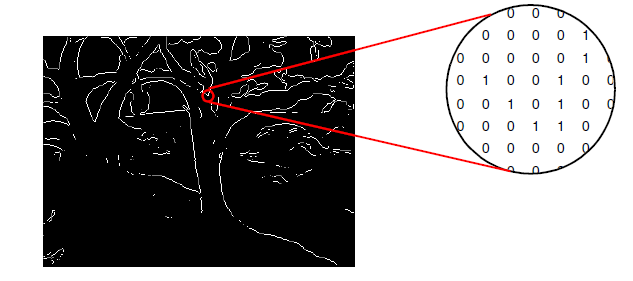Grayscale is usually the preferred format for image processing.  These are single channel images where the range for each pixel varies from 0 to 255. In cases requiring color, an RGB color image can be decomposed and handled as three separate grayscale images. Indexed images must be converted to grayscale or RGB for most operations.

Data Classes

Although we work with integer coordinates, the values of pixels themselves are not restricted to be integers in MATLAB. The given table lists the various data classes supported by MATLAB and IPT(Image Processing Toolbox) for representing pixel values. The first eight entries in the table are referred to as numeric data, the ninth entry is the char class and, as shown, the last entry is referred to as the logical data class.

All numeric computations in MATLAB are done using double quantities; this is also a frequent data class encountered in image processing applications. Class uint8 also is encountered frequently, especially when reading data from storage devices, as 8-bit images are the most common representations found in practice. These two data classes, class logical and, to a lesser degree, class uint16, constitute the primary data classes on which we focus in this book. Many IPT functions, however, support all the data classes listed . Data class double requires 8 bytes to represent a number, and uint8 and int8 require 1 byte each, and uint16 and int16 require 2 bytes, and uint32, int32 and single require 4 bytes each.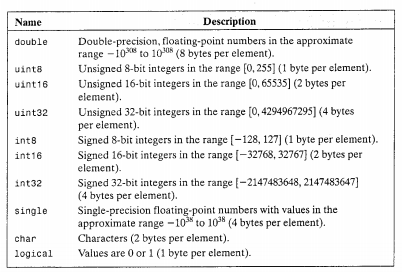The char data class holds characters in Unicode representation. A character string is merely a 1 x n array of char-acters. A array contains only the values 0 and 1, with each element being stored in memory using one byte per element. Logical arrays are created by using function logical.

Inter Conversions

A greyscale image may consist of pixels whose values are of data type uint8. These images are
thus reasonably efficient in terms of storage space, since each pixel requires only one byte. However, arithmetic operations are not permitted on this data type; a uint8 image must be converted to double before any arithmetic is attempted.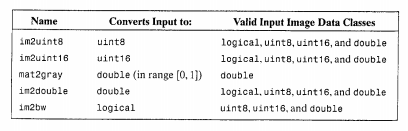We can convert images from one image type to another. Following Table lists all of Matlab’s functions for converting between different image types. Note that the gray2rgb function, does not create a colour image, but an image all of whose pixel colours were the same as before. This is done by simply replicating the grey values of each pixel: greys in an RGB image are obtained by equality of the red, green and blue values.

Function

Use

Format

ind2gray

Indexed to Greyscale

y=ind2gray(x,map);

gray2ind

Greyscale to indexed

[y,map]=gray2ind(x);

rgb2gray

RGB to greyscale

y=rgb2gray(x);

gray2rgb

Greyscale to RGB

y=gray2rgb(x);

rgb2ind

RGB to indexed

[y,map]=rgb2ind;

ind2rgb

Indexed to RGB

y=ind2rgb(x,map);